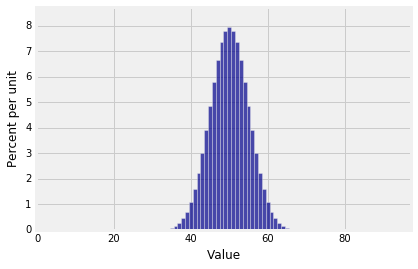## Examples¶

Here are two examples to illustrate how to find the stationary distribution and how to use it.

### A Diffusion Model by Ehrenfest¶

Paul Ehrenfest proposed a number of models for the diffusion of gas particles, one of which we will study here.

The model says that there are two containers containing a total of $N$ particles. At each instant, a container is selected at random and a particle is selected at random independently of the container. Then the selected particle is placed in the selected container; if it was already in that container, it stays there.

Let $X_n$ be the number of particles in Container 1 at time $n$. Then $X_0, X_1, \ldots$ is a Markov chain with transition probabilities given by:

\begin{equation} P(i, j) = \begin{cases} \frac{N-i}{2N} & \text{if } j = i+1 \\ \frac{1}{2} & \text{if } j = i \\ \frac{i}{2N} & \text{if } j = i-1 \\ 0 & \text{otherwise} \end{cases} \end{equation}

The chain is clearly irreducible. It is aperiodic because $P(i, i) > 0$.

Question. What is the stationary distribution of the chain?

Answer. We have computers. So let's first find the stationary distribution for $N=100$ particles, and then see if we can identify it for general $N$.

N = 100

states = np.arange(N+1)

def transition_probs(i, j):
if j == i:
return 1/2
elif j == i+1:
return (N-i)/(2*N)
elif j == i-1:
return i/(2*N)
else:
return 0

ehrenfest = MarkovChain.from_transition_function(states, transition_probs)That looks suspiciously like the binomial (100, 1/2) distribution. In fact it is the binomial (100, 1/2) distribution. Since you've guessed it, all you have to do is plug it into the balance equations and check that they work out.

The balance equations are:

\begin{align*} \pi(0) &= \frac{1}{2}\pi(0) + \frac{1}{2N}\pi(1) \\ \pi(j) &= \frac{N-(j-1)}{2N}\pi(j-1) + \frac{1}{2}\pi(j) + \frac{j+1}{2N}\pi(j+1), ~~~ 1 \le j \le N-1 \\ \pi(N) &= \frac{1}{2N}\pi(N-1) + \frac{1}{2}\pi(N) \end{align*}

You have already guessed the solution by looking at the answer calculated for $N=100$. But if you want to start from scratch, you'll have to simplify the balance equations and try to write all the elements of $\pi$ in terms of $\pi(0)$. You will get:

\begin{align*} \pi(1) &= N\pi(0) \\ \\ \pi(2) &= \frac{N(N-1)}{2} \pi0 = \binom{N}{2} \pi(0) \end{align*}

and so on by induction:

$$\pi(j) = \binom{N}{j} \pi(0)$$

In other words, the stationary distribution is proportional to the binomial coefficients. So $\pi(0) = 1/2^N$ to make all the elements sum to 1, and the distribution is binomial $(N, 1/2)$.

### Expected Reward¶

Suppose I run the lazy reflecting random walk from the previous section for a long time. As a reminder, here is its stationary distribution.

stationary = reflecting_walk.steady_state()
stationary

Value Probability
1 0.125
2 0.25
3 0.25
4 0.25
5 0.125

Question 1. Suppose that every time the chain is in state 4, I win $\$4$; every time it's in state 5, I win$\$5$; otherwise I win nothing. What is my expected long run average reward?

Answer 1. In the long run, the chain is in steady state. So I expect that on 62.5% of the moves I will win nothing; on 25% of the moves I will win $\$4$; and on 12.5% of the moves I will win$\$5$. My expected long run average reward per move is $\$1.625$. 0*0.625 + 4*0.25 + 5*.125  1.625 Question 2. Suppose that every time the chain is in state$i$, I toss$i$coins and record the number of heads. In the long run, how many heads do I expect to get on average per move? Answer 2. Each time the chain is in state$i$, I expect to get$i/2\$ heads. When the chain is in steady state, the expected number of coins I toss at any given move is 3. So, by iterated expectations, the long run average number of heads I expect to get is 1.5.

stationary.ev()/2

1.5

If that seems artificial, consider this: Suppose I play the game above, and on every move I tell you the number of heads that I get but I don't tell you which state the chain is in. I hide the underlying Markov Chain. If you try to recreate the sequence of steps that the Markov Chain took, you are working with a Hidden Markov Model. These are much used in pattern recognition, bioinformatics, and other fields.